# Ex 13.4 NCERT Solutions- Surface Areas and Volumes Class 9 Notes | EduRev

## Class 9 : Ex 13.4 NCERT Solutions- Surface Areas and Volumes Class 9 Notes | EduRev

The document Ex 13.4 NCERT Solutions- Surface Areas and Volumes Class 9 Notes | EduRev is a part of the Class 9 Course Class 9 Mathematics by VP Classes.
All you need of Class 9 at this link: Class 9

Question 1. Find the surface area of a sphere of radius: (i) 10.5 cm (ii) 5.6 cm (iii) 14 cm
Solution:
(i) Here r = 10.5 cm
∴ Surface area of the sphere = 4πr2

= 4 x (22/7) x (10.5)2 cm2

= 4 x (22/7) x (105/10) x (105/10) cm2

= 22 x 3 x 21 cm2 = 1386 cm2

(ii) Here r = 5.6 cm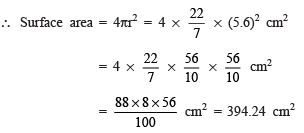(iii) Here, r = 14 cm

∴ Surface area = 4πr2 = 4 x (22/7) (14)2 cm2

= 4 x (22/7) x 14 x 14 cm2

= 4 x 22 x 2 x 14 cm2 = 2464 cm2

Question 2. Find the surface area of a sphere of diameter: (i) 14 cm (ii) 21 cm (iii) 3.5 m
Solution:
(i) Here, Diameter = 14 cm
⇒ Radius = (14/2)= 7 cm
∴ Surface area = 4πr2 = 4 x (22/7) x (7)2 cm2
= 4 x (22/7) x 7 x 7 cm2 = 88 x 7 cm= 616 cm2

(ii) Here, Diameter = 21 cm ⇒ r = (21/2) cm

∴ Surface area = 4πr2 = 4 x (22/7) (21/2)2 cm2

= 4 x (22/7) x (21/2) x (21/2) cm2

= 22 x 3 x 21 cm2 = 1386 cm2

(iii) Here, Diameter = 3.5 m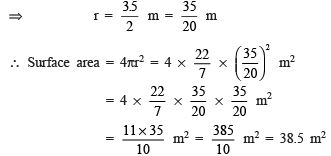Question 3. Find the total surface area of a hemisphere of radius 10 cm. (Use π = 3.14)
Solution:
Here, radius (r) = 10 cm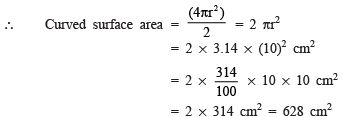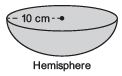Plane surface area of the hemisphere  = πr= 3.14 x (10)2 cm2

= (314/100) x 10 x 10 cm2

= 314 cm2

∴ Total surface area = 628 cm2 + 314 cm2 = 942 cm2

REMEMBER

(i) Curved surface area of a hemisphere = 2πr2
(ii) Total surface area of a hemisphere = 3πr

Question 4. The radius of a spherical balloon increases from 7 cm to 14 cm as air is being pumped into it. Find the ratio of surface areas of the balloon in the two cases.
Solution:
Case I: When radius (r1) = 7 cm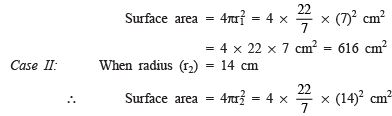= 4 x 22 x 14 x 2 cm2 = 2464 cm2
The required ratio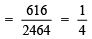⇒ The required ratio = 1 : 4

Alternate method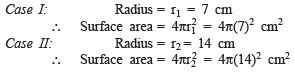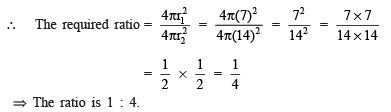Question 5. A hemispherical bowl made of brass has inner diameter 10.5 cm. Find the cost of tinplating it on the inside at the rate of ₹ 16 per 100 cm2.
Solution
: Inner diameter of the hemisphere = 10.5 m

∴    Radius (r) = 10.5/2 cm = 105/20 cm

∵ Curved surface area of a hemisphere = 2πr2
∴ Inner curved surface area of hemispherical bowl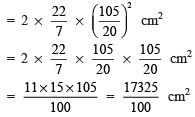Cost of tinplating: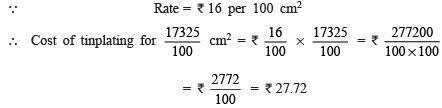Question 6. Find the radius of a sphere whose surface area is 154 cm2.
Solution:
Let the radius of the sphere be ‘r’ cm.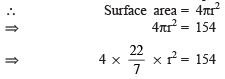∴   Surface area = 4πr2

⇒               4πr= 154

⇒              4 x 22/7 x r2 = 154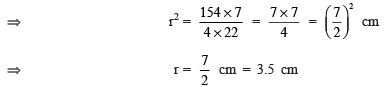Thus, the required radius of the sphere is 3.5 cm

Question 7. The diameter of the moon is apπroximately one-fourth of the diameter of the earth. Find the ratio of their surface areas.
Solution:
Let the radius of the earth = r
∴ Radius of the moon = (r/4)
∵ Surface area of a sphere = 4πr
Since, the earth as well as the moon are considered to be spheres.
∴ Surface area of the earth = 4πr2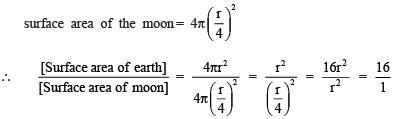or [Surface area of the moon] : [Surface area of the earth] = 1 : 16
Thus, the required ratio = 1 : 16

Question 8. A hemispherical bowl is made of steel, 0.25 cm thick. The inner radius of the bowl is 5 cm. Find the outer curved surface area of the bowl.
Solution:
Thickness = 0.25 cm
∴ Outer radius (R) = [5.00 + 0.25] cm = 5.25 cm
∴ Outer surface area of the bowl = 2πr2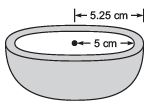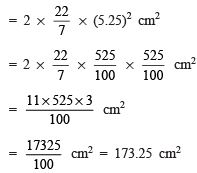Question 9. A right circular cylinder just encloses a sphere of radius r (see the figure) find (i) surface area of the sphere, (ii) curved surface area of the cylinder, (iii) ratio of the areas obtained in (i) and (ii).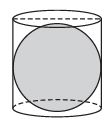Solution: (i) For the sphere: Radius = r
∴ Surface area of the sphere = 4 πr2

(ii) For the right circular cylinder:
∴ Radius of the cylinder = r
Height of the cylinder = Diameter of the sphere
⇒ Height of the cylinder (h) = 2r
Since, curved surface area of a cylinder = 2πrh = 2πr(2r) = 4πr2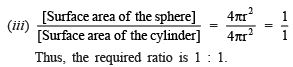VOLUME OF A CUBOID

All solid bodies occupy space. The amount of space occupied by a solid is called its volume. The units of volume are cubic centimetres (written as cm3) or cubic metres (written as m3).
Note: If an object is hollow then its interior is empty, and can be filled with fluid (air or some liquid) which will take the shape of the container. The volume of the substance that can fill the interior is called the capacity of the container.

REMEMBER

I . Volume of cuboid = [Base area] x [height]
II. Volume of the cube = Edge x Edge x Edge = (side)3

Offer running on EduRev: Apply code STAYHOME200 to get INR 200 off on our premium plan EduRev Infinity!

132 docs

,

,

,

,

,

,

,

,

,

,

,

,

,

,

,

,

,

,

,

,

,

;